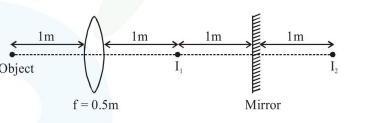# A point like object is placed at a distance of

Question:

A point like object is placed at a distance of $1 \mathrm{~m}$ in front of a convex lens of focal length $0.5 \mathrm{~m}$. A plane mirror is placed at a distance of $2 \mathrm{~m}$ behind the lens. The position and nature of the final image formed by the system is :

1. $1 \mathrm{~m}$ from the mirror, virtual

2. $1 \mathrm{~m}$ from the mirror, real

3. $2.6 \mathrm{~m}$ from the mirror, real

4. $2.6 \mathrm{~m}$ from the mirror, virtual

Correct Option: 1, 4

Solution:Object is at $2 \mathrm{f}$. So image will also be at ' $2 \mathrm{f}$ '.

$\left(\mathrm{I}_{1}\right)$

Image of $\mathrm{I}_{1}$ will be $1 \mathrm{~m}$ behind mirror.

i.e. $\Rightarrow \mathrm{I}_{2}$

Now $\mathrm{I}_{2}$ will be object for lens.

$\therefore \mathrm{u} \Rightarrow-3 \mathrm{~m}$

$\mathrm{f} \Rightarrow+0.5 \mathrm{~m}$

$\frac{1}{\mathrm{v}} \Rightarrow \frac{1}{\mathrm{f}}+\frac{1}{\mathrm{u}}$

$\Rightarrow \frac{1}{+0.5}+\frac{1}{-3}$

$\mathrm{v} \Rightarrow \frac{3}{5} \Rightarrow 0.6 \mathrm{~m}$

So total distance from mirror $\Rightarrow 2+0.6$

$\Rightarrow 2.6 \mathrm{~m}$ and real image

Ans. (3)